• ## 异或与对称差

千次阅读 2014-06-29 23:56:10
表示异或运算，用⊕表示两个集合的对称差运算。而在集合论里面用 △ 来表示对称差，在上学期学的数字逻辑里面⊕表示异或运算。这些一样的符号在不同科目里面表示不一样的运算，一样的运算在不同科目里面用不同的符号...

a在离散数学里用△表示异或运算，用⊕表示两个集合的对称差运算。而在集合论里面用△来表示对称差，在上学期学的数字逻辑里面⊕表示异或运算。这些一样的符号在不同科目里面表示不一样的运算，一样的运算在不同科目里面用不同的符号表示，这将引起极大地混乱。这个时候直觉应该告诉我们，对称差跟异或有一定的关系。它们能扯上关系吗？其实在本质上它们是一样的。或者说在布尔代数里面的异或就是在集合运算的对称差。为了比较这两种运算，下面用的讨论中，用△来表示对称差，⊕表示异或。
下面是两个运算性质的比较，这能很好的说明它们就是同一个东西，只是教科书永远都要让我们认为它们是不同的东西。
1.
首先都看看这两个运算满足什么性质。
最重要的是交换律和结合律。即
a△b=b△a，(a△b)△c=a△(b△c),
a⊕b=b⊕a,  (a⊕b)⊕c=a⊕(b⊕c);
其次是它们都满足这个性质：
a△a=∅，
a⊕a=0;
2.
a△(a△b)=b,
a⊕(a⊕b)=b
3.
如果a△b=c,那么a△c=b,b△c=a;
如果a⊕b=c,那么a⊕c=b,b⊕c=a;
就是说：如果a△b=c，那么a,b,c任何两个运算都等于剩下的那一个。
如果a⊕b=c，那么a,b,c任何两个运算都等于剩下的那一个。
4.
作为性质3的推广：
如果，那么中任何n-1个运算结果都等于剩下的那一个。
如果，那么中任何n-1个运算结果都等于剩下的那一个。（事实上，这里不一定非零即一，可以推广到任何两个正整数，异或就推广为按位异或）。
性质4，其实在我们高中的时候就已经接触过类似的东西了。那就是三角形的垂心。任何三个不共线的顶点均能连成一个三角形，这个三角形有一个垂心，垂心跟三个顶点组成四个顶点，这四个顶点中任何三个顶点连成的三角形的垂心是剩下的那个点。说得有点绕。。。意思就是这四个点中任意三个点均以第四个点为垂心。
性质4是很有用的一个运算，张金上课时就提过，数据的备份与恢复就是依据这个原理进行的。下面是这个原理：如果有n个存满数据的服务器，如何对这些数据进行backup呢？最简单的方法就是准备n个服务器，分别备份原来n个服务器的数据，但是这是很耗资源的一种方式。有没有资源节约型的方式呢？哈哈，只需要一个服务器就ok了，怎么弄呢？
把那n个服务器的数据按位异或结果按位放到这个服务器上，如果一旦其中任何一个服务器数据丢失，就可以用剩下的n个服务器的数据按位异或运算生成，这样就实现了一台机器对多台机器的数据进行备份。当然了，如果有两个服务器或者更多的服务器数据丢失的话，这种方法就没辙了。通常这种情况不会发生，只要把这些服务器在地理上分隔，一般的大灾难蔓延的距离就几百公里。。两个相隔较远的地方的服务器同时出事的可能性太小了。因此，可以通过这样的方式备份。这也是数学应用于生活的一个例子。

展开全文数学 数据 服务器 备份
• STL库中有丰富的集合运算方法，我们可以使用它们快速完成交集、并集、差集、对称差集的运算。（转载请指明出于breaksoftware的csdn博客） 交集（intersection） 交集是集合运算中经常会用到的计算，其表达是两个...
        STL库中有丰富的集合运算方法，我们可以使用它们快速完成交集、并集、差集、对称差集的运算。（转载请指明出于breaksoftware的csdn博客）

交集（intersection）

交集是集合运算中经常会用到的计算，其表达是两个集合共有的部分（图中红色区域）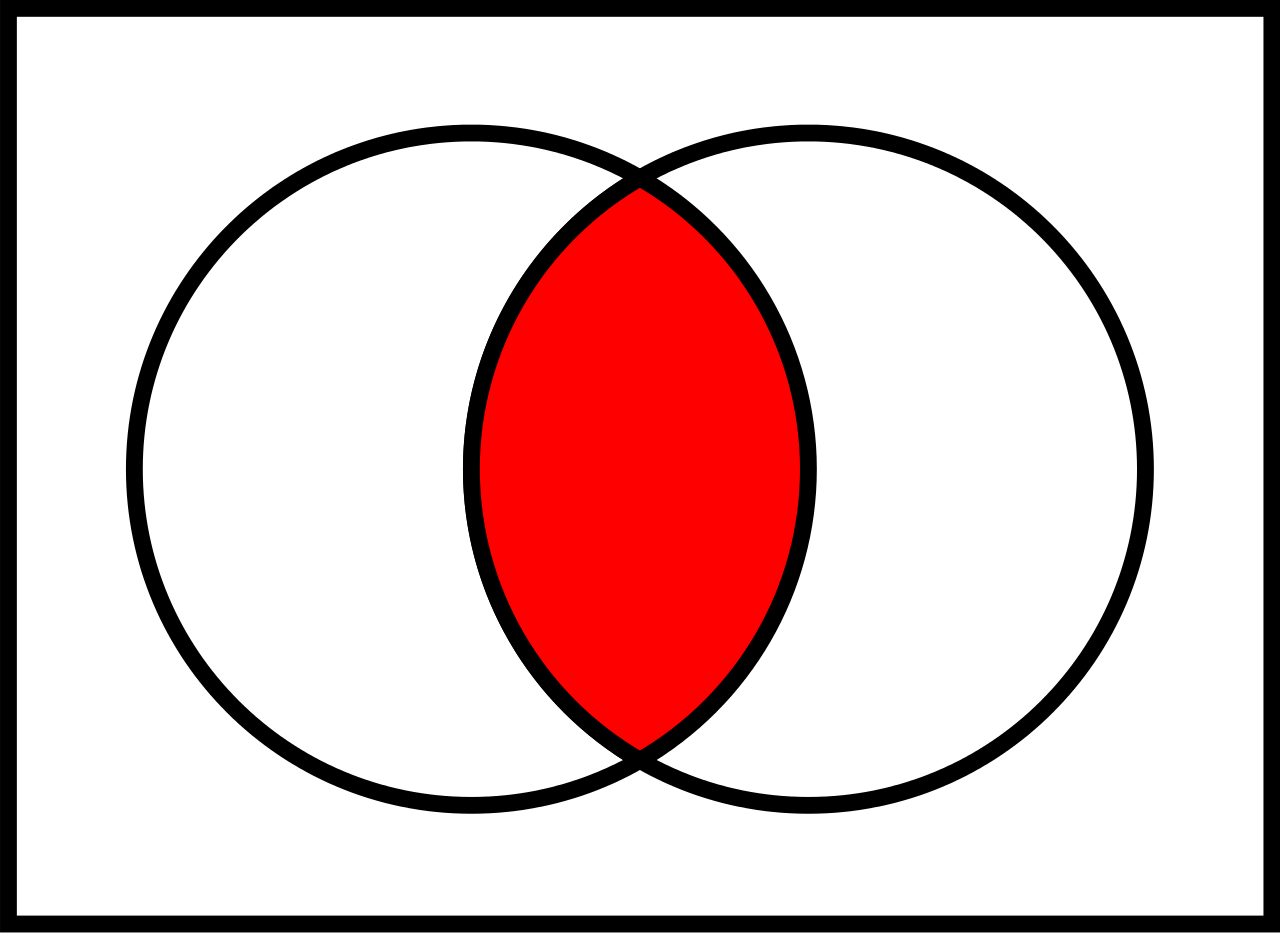STL中有set_intersection方法可以实现该功能。它是C++17开始支持的方法，声明于<algorithm>中。其中一种形式是

template< class ExecutionPolicy, class ForwardIt1, class ForwardIt2, class ForwardIt3 >
ForwardIt3 set_intersection( ExecutionPolicy&& policy, ForwardIt1 first1, ForwardIt1 last1,
ForwardIt2 first2, ForwardIt2 last2,
ForwardIt3 d_first );


第一二个参数是某个集合的起止迭代器，第二三个参数是另一个集合的起止迭代器。这两个待比较集合要求是有序的。最终得到的交集保存在第五个参数所指向的集合的起始迭代器位置。

我们看个例子

#include <vector>
#include <iostream>
#include <iterator>
#include <algorithm>

int main() {
std::vector<int> a{1, 3, 3, 4, 4, 5, 6};
std::vector<int> b{2, 3, 4, 4, 5, 5, 7};
std::sort(a.begin(), a.end());
std::sort(b.begin(), b.end());

std::vector<int> result;

std::set_intersection(a.begin(), a.end(),
b.begin(), b.end(),
std::back_inserter(result));

std::copy(result.begin(), result.end(),
std::ostream_iterator<int>(std::cout, " "));
return 0;
}

第7~10行保证了待比较的两个集合是有序的。第14行是将a、b两个集合的交集保存到result集合中。最终输出的是

3 4 4 5 

并集（union）

并集是指两个集合组合在一起集合（图中红色区域）。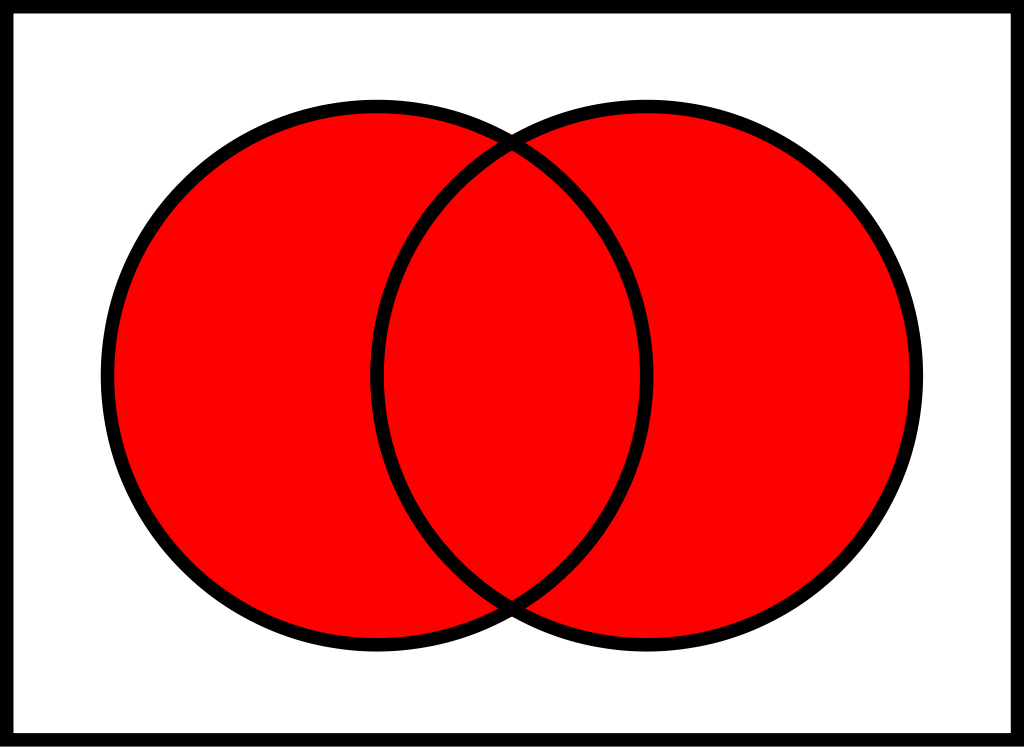set_union

STL中有set_union方法可以实现该功能。它是C++17开始支持的方法，声明于<algorithm>中。其中一种形式是

template< class ExecutionPolicy, class ForwardIt1, class ForwardIt2, class ForwardIt3 >
ForwardIt3 set_union( ExecutionPolicy&& policy, ForwardIt1 first1, ForwardIt1 last1,
ForwardIt2 first2, ForwardIt2 last2,
ForwardIt3 d_first );

它的第一二个参数是某个集合的起止迭代器，第二三个参数是另一个集合的起止迭代器。这两个待合并集合要求是有序的。最终得到的并集保存在第五个参数所指向的集合的起始迭代器位置。

我们看个例子

#include <vector>
#include <iostream>
#include <iterator>
#include <algorithm>

int main() {
std::vector<int> a{ 1, 3, 3, 4, 4, 5, 6 };
std::vector<int> b{ 2, 3, 4, 4, 5, 5, 7 };
std::sort(a.begin(), a.end());
std::sort(b.begin(), b.end());

std::vector<int> result;

std::set_union(a.begin(), a.end(),
b.begin(), b.end(),
std::back_inserter(result));

std::copy(result.begin(), result.end(),
std::ostream_iterator<int>(std::cout, " "));
return 0;
}

其结果是

1 2 3 3 4 4 5 5 6 7

a集合有2个3，b集合有1个3。理论上应该有3个3，但是set_union方法只从取max(countof(a, 3),  countof(b, 3))个元素，所以只有2个3。如果希望取到3个3的并集，可以使用merge方法

merge

C++17开始支持该方法，其定义于<algorithm>。它的一种形式是

template< class InputIt1, class InputIt2, class OutputIt >
constexpr OutputIt merge( InputIt1 first1, InputIt1 last1,
InputIt2 first2, InputIt2 last2,
OutputIt d_first );

它的第一二个参数是某个集合的起止迭代器，第二三个参数是另一个集合的起止迭代器。这两个待合并集合要求是有序的。最终得到的并集保存在第五个参数所指向的集合的起始迭代器位置。

将上例中set_union改成merge方法后，输出结果是

1 2 3 3 3 4 4 4 4 5 5 5 6 7

差集（difference）

差集是指在一个集合中，不再另外一个集合中的部分（图中红色区域）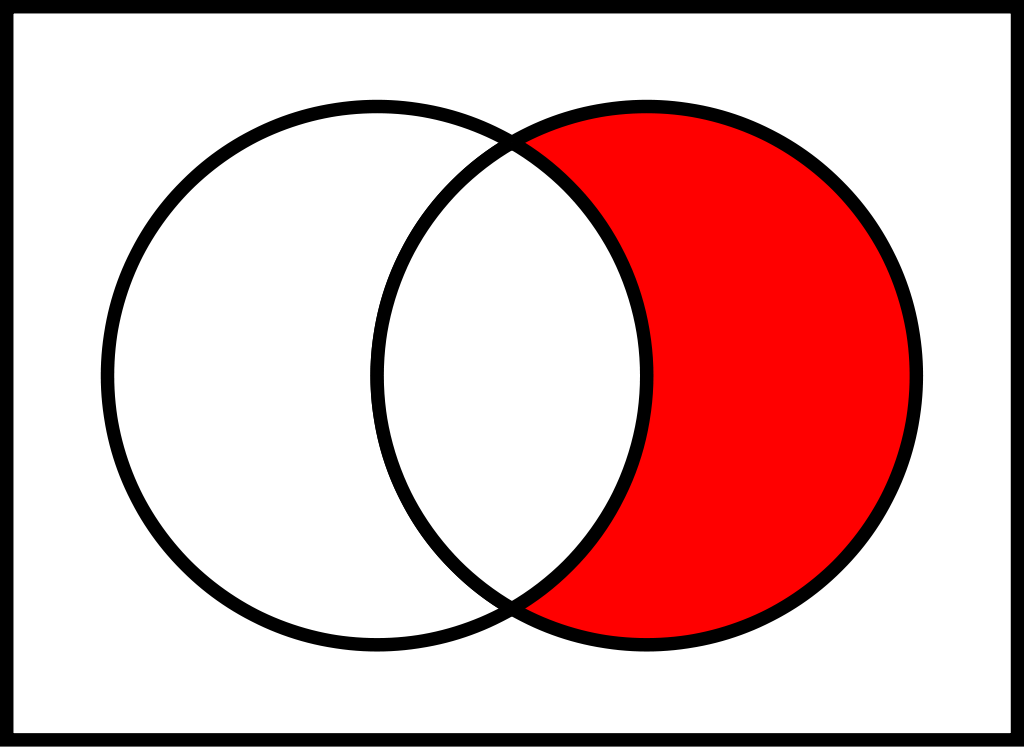可以见得，两个集合的差集存在两个可能性：一种是在左侧集合不在右侧集合中的部分；一种是在右侧集合不在左侧集合中的部分。

STL中有set_difference方法可以实现该功能。它是C++17开始支持的方法，声明于<algorithm>中。其中一种形式是

template< class InputIt1, class InputIt2, class OutputIt >
OutputIt set_difference( InputIt1 first1, InputIt1 last1,
InputIt2 first2, InputIt2 last2,
OutputIt d_first );

它的第一二个参数是左侧集合的起止迭代器，第二三个参数是右侧集合的起止迭代器。这两个待比较集合要求是有序的。最终得到的差集保存在第五个参数所指向的集合的起始迭代器位置。

我们看个例子

#include <vector>
#include <iostream>
#include <iterator>
#include <algorithm>

int main() {
std::vector<int> a{ 1, 3, 3, 4, 4, 5, 6 };
std::vector<int> b{ 2, 3, 4, 4, 5, 5, 7 };
std::sort(a.begin(), a.end());
std::sort(b.begin(), b.end());

std::vector<int> result;

std::set_difference(a.begin(), a.end(),
b.begin(), b.end(),
std::back_inserter(result));

std::copy(result.begin(), result.end(),
std::ostream_iterator<int>(std::cout, " "));
return 0;
}

这个例子中，我们将算出在a集合中，不在b集合中的元素，并保存到result中。其结果是

1 3 6

由于a集合中有两个3，所以结果中有一个3。

如果求在集合b中，不在集合a中的集合，只需要把std::set_difference中a、b替换位置

    std::set_difference(b.begin(), b.end(),
a.begin(), a.end(),
std::back_inserter(result));

得出的结果是

2 5 7

对称差（symmetric difference）

对称差是指并集中，去除交集之外的部分（图中红色区域）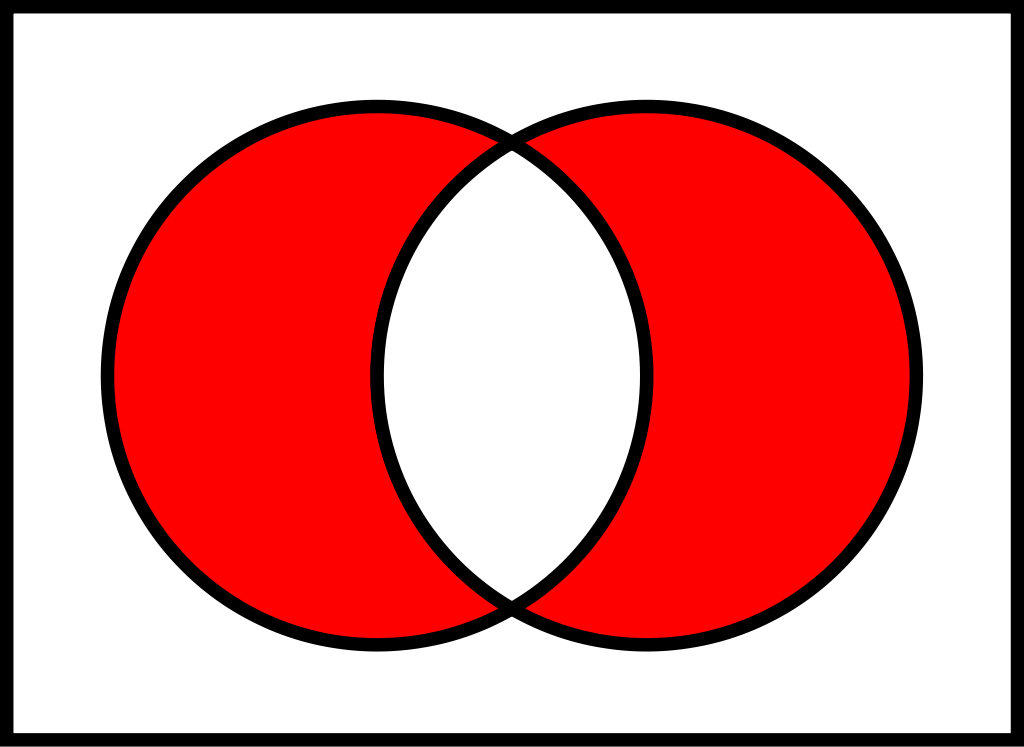STL中有set_symmetric_difference方法可以实现该功能。它是C++17开始支持的方法，声明于<algorithm>中。其中一种形式是

template< class InputIt1, class InputIt2, class OutputIt >
OutputIt set_symmetric_difference( InputIt1 first1, InputIt1 last1,
InputIt2 first2, InputIt2 last2,
OutputIt d_first );

它的第一二个参数是某个集合的起止迭代器，第二三个参数是另一个集合的起止迭代器。这两个待比较集合要求是有序的。最终得到的等差分集保存在第五个参数所指向的集合的起始迭代器位置。

我们看个例子

#include <vector>
#include <iostream>
#include <iterator>
#include <algorithm>

int main() {
std::vector<int> a{ 1, 3, 3, 4, 4, 5, 6 };
std::vector<int> b{ 2, 3, 4, 4, 5, 5, 7 };
std::sort(a.begin(), a.end());
std::sort(b.begin(), b.end());

std::vector<int> result;

std::set_symmetric_difference(a.begin(), a.end(),
b.begin(), b.end(),
std::back_inserter(result));

std::copy(result.begin(), result.end(),
std::ostream_iterator<int>(std::cout, " "));
return 0;
}

得到的结果是

1 2 3 5 6 7 

在项目中，我们使用上述集合操作方法，将使代码变的简洁。

展开全文C++ STL 集合算法
• } /*The difference has 3 elements: 5 15 25 */ 对称差 // set_symmetric_difference example #include // cout #include // set_symmetric_difference, std::sort #include // vector using namespace std;...
交集
// set_intersection example
#include <iostream>     // cout
#include <algorithm>    // set_intersection, sort
#include <vector>       // vector
using namespace std;
int main () {
int first[] = {5,10,15,20,25};
int second[] = {50,40,30,20,10};
vector<int> v(10);                      // 0  0  0  0  0  0  0  0  0  0
vector<int>::iterator it;

sort (first,first+5);     //  5 10 15 20 25
sort (second,second+5);   // 10 20 30 40 50

it=set_intersection (first, first+5, second, second+5, v.begin());
// 10 20 0  0  0  0  0  0  0  0
v.resize(it-v.begin());                      // 10 20

cout << "The intersection has " << (v.size()) << " elements:\n";
for (it=v.begin(); it!=v.end(); ++it)
cout << ' ' << *it;
cout << '\n';

return 0;
}/*The intersection has 2 elements:
10 20
*/

并集
// set_union example
#include <iostream>     // cout
#include <algorithm>    // set_union, sort
#include <vector>       // vector
using namespace std;
int main () {
int first[] = {5,10,15,20,25};
int second[] = {50,40,30,20,10};
vector<int> v(10);                      // 0  0  0  0  0  0  0  0  0  0
vector<int>::iterator it;

//要用交集函数必须先排序
sort (first,first+5);     //  5 10 15 20 25
sort (second,second+5);   // 10 20 30 40 50

it=set_union (first, first+5, second, second+5, v.begin());
// 5 10 15 20 25 30 40 50  0  0
v.resize(it-v.begin());                      // 5 10 15 20 25 30 40 50

cout << "The union has " << (v.size()) << " elements:\n";
for (it=v.begin(); it!=v.end(); ++it)
cout << ' ' << *it;
cout << '\n';

return 0;
}
/*The union has 8 elements:
5 10 15 20 25 30 40 50
*/

差集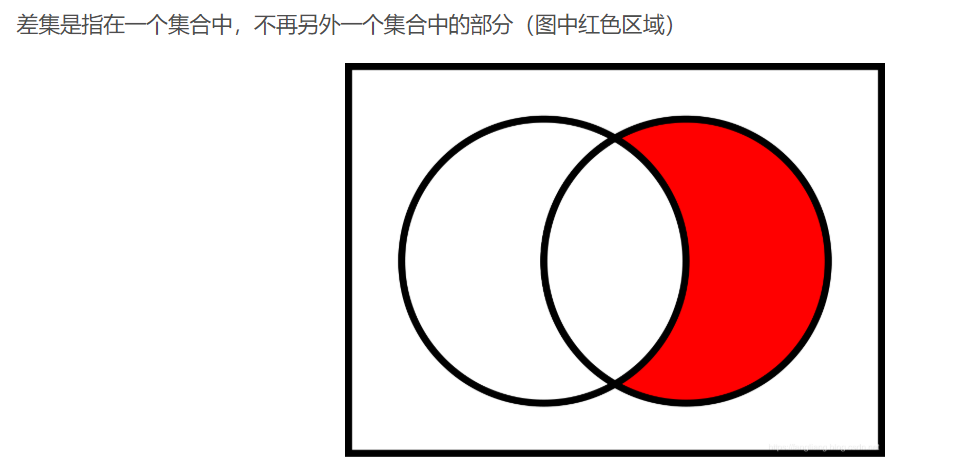// set_difference example
#include <iostream>     // cout
#include <algorithm>    // set_difference, sort
#include <vector>       // vector
using namespace std;
int main () {
int first[] = {5,10,15,20,25};
int second[] = {50,40,30,20,10};
vector<int> v(10);                      // 0  0  0  0  0  0  0  0  0  0
vector<int>::iterator it;

sort (first,first+5);     //  5 10 15 20 25
sort (second,second+5);   // 10 20 30 40 50

it=set_difference (first, first+5, second, second+5, v.begin());
//  5 15 25  0  0  0  0  0  0  0
v.resize(it-v.begin());                      //  5 15 25

cout << "The difference has " << (v.size()) << " elements:\n";
for (it=v.begin(); it!=v.end(); ++it)
cout << ' ' << *it;
cout << '\n';

return 0;
}
/*The difference has 3 elements:
5 15 25
*/

对称差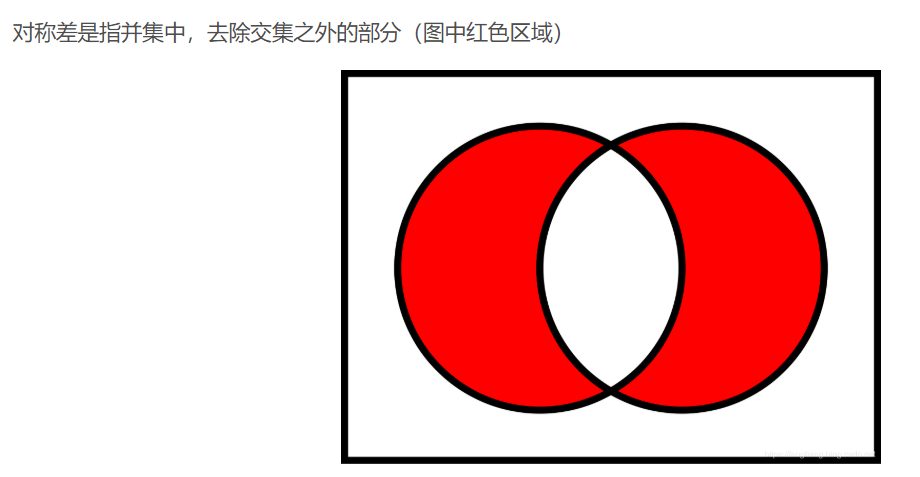// set_symmetric_difference example
#include <iostream>     // cout
#include <algorithm>    // set_symmetric_difference, std::sort
#include <vector>       // vector
using namespace std;
int main () {
int first[] = {5,10,15,20,25};
int second[] = {50,40,30,20,10};
vector<int> v(10);                      // 0  0  0  0  0  0  0  0  0  0
vector<int>::iterator it;

sort (first,first+5);     //  5 10 15 20 25
sort (second,second+5);   // 10 20 30 40 50

it=set_symmetric_difference (first, first+5, second, second+5, v.begin());
//  5 15 25 30 40 50  0  0  0  0
v.resize(it-v.begin());                      //  5 15 25 30 40 50

cout << "The symmetric difference has " << (v.size()) << " elements:\n";
for (it=v.begin(); it!=v.end(); ++it)
cout << ' ' << *it;
cout << '\n';

return 0;

}
/*The symmetric difference has 6 elements:
5 15 25 30 40 50*/




展开全文• 编写程序实现两个集 合的并、交，相对补，对称差，幂集的运算并判断两个集合是否相等

任务描述

编写程序实现两个集 合的并、交，相对补，对称差的运算并判断两个集合是否相等?

功能要求

①输入集合A与集合B的元素。

②输出集合A与B的并、交、相对补、差、对称差运算。

③输出集合A与B是否相等的断定结果。

④输出集合A与B的幂集。

//这个程序的幂集计算有错，做了修改，在另外一篇文章

http://blog.csdn.net/lu_1u/article/details/73864012

#include<stdio.h>
#include<stdlib.h>

int unionSets(int *A, int *B, int lenA, int lenB)//并集
{
int i, j;
int flag, index = lenB;
int *buffer = (int*)malloc(sizeof(int) * (lenA + lenB));
for (i = 0; i < lenB; i++) {
buffer[i] = B[i];
}
for (i = 0; i < lenA; i++) {
flag = 1;
for (j = 0; j < lenB; j++) {
if (A[i] == B[j]) {
flag = 0;
}
}
if (flag) {
buffer[index] = A[i];
index++;
}
}
printf("并集:{ ");
for (i = 0; i < index; i++) {
printf("%d ", buffer[i]);
}
printf("}\n");
}

int intersection(int *A, int *B, int lenA, int lenB)//交集
{
int i, j;
int flag, index = 0, len = lenA > lenB ? lenA : lenB;
int *buffer = (int*)malloc(sizeof(int) * len);
for (i = 0; i < lenA; i++) {
flag = 0;
for (j = 0; j < lenB; j++) {
if (A[i] == B[j]) {
flag = 1;
}
}
for (j = 0; j < index; j++) {
if (A[i] == buffer[j]) {
flag = 0;
}
}
if (flag) {
buffer[index] = A[i];
index++;
}
}
printf("交集:{ ");
for (i = 0; i < index; i++) {
printf("%d ", buffer[i]);
}
printf("}\n");

}

int complementary(int *A, int *B, int lenA, int lenB)//相对补
{
int i, j;
int flag, index = 0;
int *buffer = (int*)malloc(sizeof(int)*lenA);
for (i = 0; i < lenA; i++) {
flag = 1;
for (j = 0; j < lenB; j++) {
if (A[i] == B[j]) {
flag = 0;
}
}
if (flag) {
buffer[index] = A[i];
index++;
}
}
printf("相对补:{ ");
for (i = 0; i < index; i++) {
printf("%d ", buffer[i]);
}
printf("}\n");
}

int SymDifference(int *A, int *B, int lenA, int lenB)//对称差
{
int i, j;
int flag, index = 0;
int *buffer = (int*)malloc(sizeof(int)*lenA);
for (i = 0; i < lenA; i++) {
flag = 1;
for (j = 0; j < lenB; j++) {
if (A[i] == B[j]) {
flag = 0;
}
}
if (flag) {
buffer[index] = A[i];
index++;
}
}
for (i = 0; i < lenB; i++) {
flag = 1;
for (j = 0; j < lenA; j++) {
if (B[i] == A[j]) {
flag = 0;
}
}
if (flag) {
buffer[index] = B[i];
index++;
}
}
printf("对称差:{ ");
for (i = 0; i < index; i++) {
printf("%d ", buffer[i]);
}
printf("}\n");
}

int equalSets(int *A, int *B, int lenA, int lenB)//集合相等
{
int top = 0, i, j, temp;
int *buffer = (int*)malloc(sizeof(int) * lenA);
if (lenA != lenB) {
printf("\nA与B相等\n");
return 0;
}
for (i = 0; i < lenA; i++) {
for (j = i + 1; j < lenA; j++) {
if (A[i] < A[j]) {
temp = A[i];
A[i] = A[j];
A[j] = temp;
}
}
}
for (i = 0; i < lenB; i++) {
for (j = i + 1; j < lenB; j++) {
if (B[i] < B[j]) {
temp = B[i];
B[i] = B[j];
B[j] = temp;
}
}
}
for (i = 0; i < lenA; i++) {
if (A[i] != B[i]) {
printf("\nA与B不相等\n");
return 0;
}
}
printf("相等\n");
}

int powerSets(int *A, int lenA)//幂集
{
int i, j;
int tag, index;
printf("幂集:{ { 空集 }, ");
for (tag = 1; tag <= lenA; tag++) {
for (index = 0; index < lenA - tag + 1; index++) {
printf("{ ");
for (i = index,j=0; j < tag; i++,j++) {
printf("%d ", A[i]);
}
printf("},");
}
}
printf("}\n");
}

int main()
{
int *A, *B;
int lenA, lenB;
int i;
printf("输入A,B的元素个数:  ");
scanf("%d%d", &lenA, &lenB);

A = (int*)malloc(sizeof(int) * lenA);
B = (int*)malloc(sizeof(int) * lenB);
printf("输入A的元素\n");
for (i = 0; i < lenA; i++) {
scanf("%d", &A[i]);
}
getchar();
printf("输入B的元素\n");
for (i = 0; i < lenB; i++) {
scanf("%d", &B[i]);
}
equalSets(A, B, lenA, lenB);
unionSets(A, B, lenA, lenB);
intersection(A, B, lenA, lenB);
printf("A对B的");
complementary(A, B, lenA, lenB);
printf("B对A的");
complementary(B, A, lenA, lenB);
SymDifference(A, B, lenA, lenB);
printf("A的");
powerSets(A, lenA);
printf("B的");
powerSets(B, lenB);
system("pause");
return 0;
}

展开全文c语言 模块化 集合运算
• } /** 交、差、并、对称差运算都是用到了algorithm函数库中的函数 这四个函数的语法都是通用的： 以set_union为例： set_union(data.begin(),data.end(),anotherSets.data.begin(),anotherSets.data.end(), ...c++ 算法 编程语言
• 针对它们做的最多的操作就是求交集,并集,差集,对称差集.不过集合有个特性就是不能有重复的元素. 而STL中的算法中的交并集,所用到的容器不一定要是不能有重复元素.并集等的结果是排好序的一个集合.默认是通过容器的...
• ## 对数函数

万次阅读 2019-11-10 22:27:37
一般地，对数函数是以幂（真数）为自变量，指数为因变量，底数为常量的函数。 对数函数是6类基本初等函数之一。其中对数的定义： 如果ax=N（a>0，且a≠1），那么数x叫做以a为底N的对数，记作x=logaN，读作以a为...
• 数学上，两个集合的对称差集是只属于其中一个集合，而不属于另一个集合的元素组成的集合。也就是说对称差集是去除同时出现在两个集合中的元素后，两集合其他元素组成的集合。例如：集合{1,2,3,7,9}和{3,4,5,7}的对称...
• 通过algorithm中提供的set_intersection、set_union、set_difference、set_symmetric_difference四个函数，可以方便的实现集合的交、并、差、对称差操作，很实用！ 微软帮助文档中对集合（set）的解释： “描述...stl c++ set 集合操作
• 数学上，两个集合的对称差集是只属于其中一个集合，而不属于另一个集合的元素组成的集合。也就是说对称差集是去除同时出现在两个集合中的元素后，两集合其他元素组成的集合。例如：集合{1,2,3,7,9}和{3,4,5,7}的对称...
• ## 势函数

万次阅读 2017-03-24 09:34:32
• ## 核函数

万次阅读 2012-06-04 11:08:02
函数   (2010-12-23 23:08:30) ...高斯核函数 ... Basis Function 简称 RBF), 就是某种沿径向对称的标量函数。通常定义为空间中任一点x到某一中心xc之间欧氏距离的单调函数 , 可记作 k(||x-xc||),优化 算法 plot methods vector
• 文章目录第1章： 图像显示与图像文件输入输出函数第2章： 图形绘制第3章： 图像类型和类型转换第4章： 图形用户界面工具第5章： 空间变换和图像配准第6章： 图像分析和统计第7章： 图像代数运算第8章： 图像增强第9...matlab 图像处理
• 用势函数的概念来确定判别函数和划分类别界面。 基本思想 假设要划分属于两种类别ω1和ω2的模式样本，这些样本可看成是分布在n维模式空间中的点xk。把属于ω1的点比拟为某种能源点，在点上，电位达到峰值。随着...机器学习 数据
• ## Matlab常用函数

千次阅读 2014-06-03 10:15:00
Matlab有没有求矩阵行数/列数/维数的函数？ ndims(A)返回A的维数 size(A)返回A各个维的最大元素个数 length(A)返回max(size(A)) [m,n]=size(A)如果A是二维数组，返回行数和列数 nnz(A)返回A中非0元素的个数 ...
• MATLAB图像处理中关于自定义双峰函数twomodegauss的应用。matlab
• ## matlab常用函数

千次阅读 2019-09-07 16:33:57
一、软件操作函数 1）命令窗口函数： clc：清空命令窗口，使用向上箭头翻看命令。 open:打开文件，文本文件（*.doc），可执行文件（*.exe），图形文件（*.fig），超文本文件（*.html，*.htm），MATLAB数据库文件（*....matlab
• 1.核函数 1.1核函数的由来 -----------还记得为何要选用核函数么？----------- 对于这个问题，在Jasper's Java Jacal博客《SVM入门（七）为何需要核函数》中做了很详细的阐述，另外博主对于SVM德入门学习也是做了很...svm
• ## matlab函数总结

千次阅读 2018-07-28 10:38:58
ndims(A)返回A的维数 size(A)返回A各个维的最大元素个数 length(A)返回max(size(A)) [m,n]=size(A)如果A是二维数组，返回行数和列数 nnz(A)返回A中非0元素的...MATLAB的取整函数:fix(x), floor(x) :,ceil(x) , ro...matlab
• 数字摘要和Hash函数（哈希函数） 1、数字摘要 数字摘要是将任意长度的消息变成固定长度的短消息，它类似于一个自变量是消息的函数，也就是Hash函数。数字摘要就是采用单项Hash函数将...一般来说，非对称加密是用来处理
• imfilter函数： 下面这段是转的翻译： 用法：B = imfilter(A,H) B = imfilter(A,H,option1,option2,...) 或写作g = imfilter(f, w, filtering_mode, boundary_options, size_options) 其中，f为输入图像，w...
• 文章目录一、两种分布1.1 高斯分布（正态分布）1.2 柯西分布（洛伦兹分布）二、光谱线型函数2.1 光谱线型函数的几种形式2.2 洛伦兹线型函数与多普勒（高斯）线型函数2.3 vogit 线型函数参考文章 一、两种分布 1.1 ...
• ## Sigmoid函数总结

千次阅读 2019-02-22 20:35:49
以前一直不是很理解Sigmoid函数的重要性，查了很多资料，大体上屡了一下，不过数学功底太，很多地方理解的不够透彻。这里简单记录一下。 一 函数基本性质 二 Sigmoid函数与逻辑回归 三 为什么要选择Sigmoid函数 ...
• ## MATLAB函数速查手册

千次阅读 多人点赞 2018-03-25 09:06:26matlab
• set（）函数语法 集合类型操作符 标准类型操作符(所有) 成员关系 in 和not in 集合等价/不等价 == ！= 子集/超集 < <= > >= 集合类型操作符(所有) 集合类型操作符(仅适用于可变集合) 内建函数 ...
• ## 散列函数

千次阅读 2010-03-31 07:34:00
[转载]散列函数· 散列函数（或散列算法，英语：Hash Function）是一种从任何一种数据中创建小的数字“指纹”的方法。该函数将数据打乱混合，重新创建一个叫做散列值的指纹。散列值通常用来代表一个短的随机字母和...算法 加密 数据库 filematlab编程
• ## 高斯核函数

千次阅读 2019-07-07 16:45:53
1.二维高斯函数形式 A是幅值，（xo,y0）为中心点坐标，σxσy是方差，图示如下，A=1,(X0,Y0)=(0,0),σx= σy= 1 2.高斯函数分析 在实际编程应用中，高斯函数的参数包括-- ksize -- 高斯函数的大小 sigma --...
• 三角函数的性质 一系列三角函数谐波（harmonic sinusoids）是傅里叶分析的基石，我们可以用这些不同频率的谐波构建各种各样的信号/波形。 谐波（harmonics）： 现在我们选择一个频率为f0的任意频率...Gibbs...